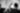# Seminar: Gergely Neu - Pompeu Fabra University

Date May 21, 2020
Author Hrvoje Stojic

## Abstract

We propose a general framework for entropy-regularized average-reward reinforcement learning in Markov decision processes (MDPs). Our approach is based on extending the linear-programming formulation of policy optimization in MDPs to accommodate convex regularization functions. Our key result is showing that using the conditional entropy of the joint state-action distributions as regularization yields a dual optimization problem closely resembling the Bellman optimality equations. This result enables us to formalize a number of state-of-the-art entropy-regularized reinforcement learning algorithms as approximate variants of Mirror Descent or Dual Averaging, and thus to argue about the convergence properties of these methods. In particular, we show that the exact version of the TRPO algorithm of Schulman et al. (2015) actually converges to the optimal policy, while the entropy-regularized policy gradient methods of Mnih et al. (2016) may fail to converge to a fixed point. Finally, we illustrate empirically the effects of using various regularization techniques on learning performance in a simple reinforcement learning setup.

### Notes

• ArXiV preprint can be found here
• Gergely Neu is a Research Assistant Professor, AI group, DTIC, Universitat Pompeu Fabra. His personal website can be found here .
Share
,,
Optimization Engine